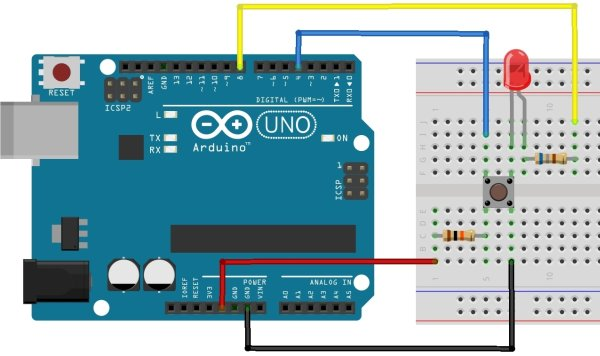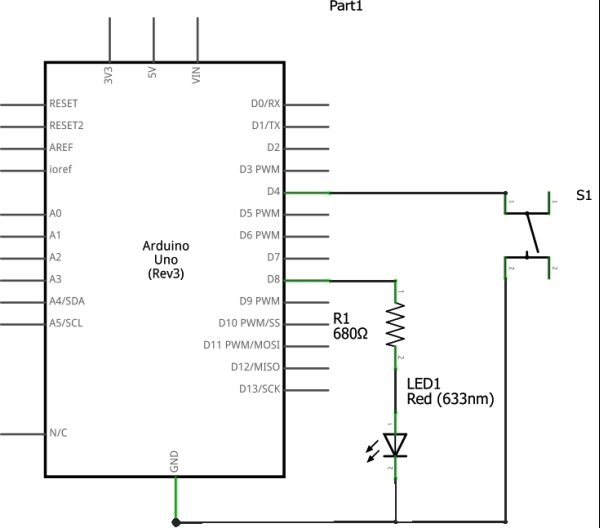# Using Push Button Switch with Arduino Uno

In this tutorial you will learn how to read the status of a digital pin of Arduino. I hope that you already go through our first tutorial, Getting Started with Arduino Uno – LED Blinking. In this example, a push button switch and an LED is connected to Arduino Uno. When we press the switch, LED will glow for 3 seconds. A pin is configured as Input Pin to connect switch and another pin is configured as Output Pin to connect LED. You need to connect PULL-UP or PULL-DOWN resistors while interfacing switch.If we haven’t use PULL-UP or PULL-DOWN resistors, there will be an UNDETERMINED STATE (neither LOW nor HIGH) when the switch is OPEN. In this tutorial we will follow PULL-UP resistors.

Digital Pins of Arduino can be configured as OUTPUT, INPUT or INPUT_PULLUP mode using pinMode() function. INPUT_PULLUP mode is used to enable the Internal PULL-UP Resistor. The value of Internal PULL-UP resistor of Arduino Uno is about 20-50KΩ.

Now we have two options to interface switches with Arduino :

1. Using External PULL-UP Resistor
2. Using Internal PULL-UP Resistor

The simple solution is to using Internal PULL-UP resistor but in some cases you may have to use External PULL-UP resistors.

# Using External PULL-UP Resistor

This method is not recommended, but it is explained first just because it is easy to understand. In some cases you may forced to use External PULL-UP resistor.

## Circuit Diagram

Here the switch is connected to digital pin 4 (D4) and LED is connected to digital pin 8 (D8) of Arduino Uno. Thus pin D4 should be declared as INPUT and pin D8 as OUTPUT in the sketch (program). 10KΩ resistor is used as PULL-UP resistor and a 680Ω resistor is used to limit the current through the LED.

## Arduino Sketch

```const int switchPin = 4; //Switch Connected to PIN 4
const int ledPin = 8; //LED Connected to PIN 8
int switchState = 0; // Variable for reading Switch status
void setup()
{
pinMode(ledPin, OUTPUT); //LED PIN is Output
pinMode(switchPin, INPUT);//Switch PIN is input with PULLUP
}
void loop()
{
if (switchState == LOW) //If the switch is pressed
{
digitalWrite(ledPin, HIGH); //LED ON
delay(3000); //3 Second Delay
digitalWrite(ledPin, LOW); //LED OFF
}
}```When the switch is off, pin D4 will be LOGIC HIGH state due to the action of PULL-UP resistor. Whenever the switch is pressed, pin D4 will switch to LOGIC LOW state since the closed switch will ground the pin. In the above sketch setup() funtion will run initially and sets pin modes. Then the loop() function will run as an infinite loop, which checks the status of the switch continuously. Whenever the switch press is detected, LED is turned ON and it is turned OFF after a 3 seconds delay.

For more detail: Using Push Button Switch with Arduino Uno

#### This Post / Project can also be found using search terms:

• led control with switch in arduino uno
• Arduino uno switch case button
• using switches with arduino
• arduino input/output using push button